/
/
/
91) Use the following MRP record to answer the following
Not my Question
Flag Content

# Question : 91) Use the following MRP record to answer the following : 2045337

91) Use the following MRP record to answer the following question.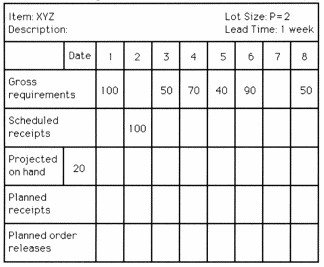What is the planned order release quantity in week 4?

A) 0 units

B) greater than 0 units but less than or equal to 50 units

C) greater than 50 units but less than or equal to 100 units

D) greater than 100 units

Table 13.1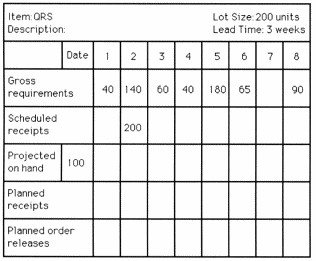92) Use the information in Table 13.1. In which week will the second planned order release occur?

A) Week 1

B) Week 2

C) Week 3

D) Week 4, 5, 6, 7 or 8

93) Use the information in Table 13.1. If the safety stock level were 30 units instead of 0 units, in which week would the first planned receipt occur?

A) Week 1

B) Week 2 or 3

C) Week 4 or 5

D) Week 6 or 7

94) Use the following MRP record to answer the question.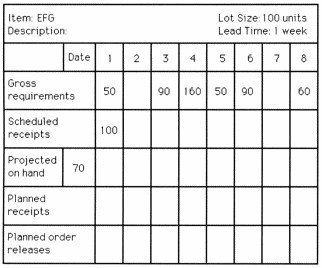What will be the projected on-hand inventory quantity in week 8?

A) less than or equal to 30 units

B) greater than 30 units but less than or equal to 60 units

C) greater than 60 units but less than or equal to 90 units

D) greater than 90 units

95) Use the following MRP record to answer the question.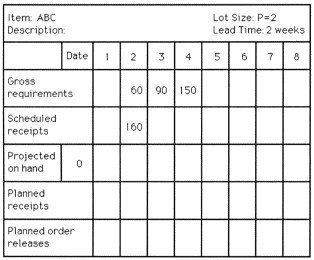What are the quantity and the week of the first planned order release?

A) 20 units; week 1

B) 140 units; week 2

C) 150 units; week 1

Table 13.2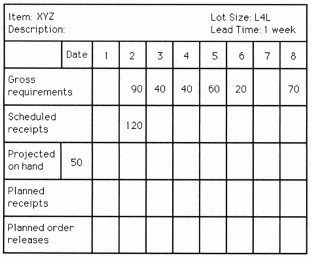96) Use the information in Table 13.2. How many planned order releases are there for item XYZ?

A) 0

B) 1 or 2

C) 3

D) 4 or more

97) Use the information in Table 13.2. If the lot sizing was POQ (P = 3) instead of L4L, how many planned order releases would there be?

A) 0

B) 1 or 2

C) 3

D) 4 or more

98) Use the following MRP record to answer the question.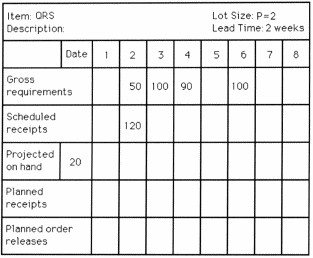Which one of the following statements about item QRS is TRUE?

A) Exactly 1 action notice is needed.

B) The first planned receipt occurs in week 1.

C) The second planned order release quantity is 90 units.

D) The total planned order release quantity (summing up all 8 weeks) is 190 units.

99) Use the following MRP record to answer the question.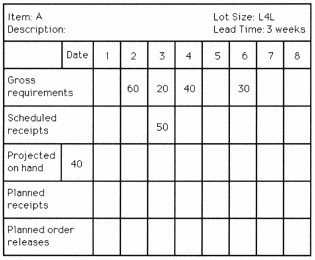How many action notices are needed for item A?

A) 0

B) 1

C) 2

D) 3 or more

Table 13.3

Consider the following MPS, BOM, and inventory data. You will need a blank MRP record to answer the question(s).

Master Production Schedule: the following table shows the MPS start quantities.

Week

 Week 1 2 3 4 5 6 A B 50 80 60 100 100

Bill of Material

-End item A uses 1 each of component C and 1 each of subassembly D.

-Subassembly D uses 2 each of component E and 2 each of component F.

-End item B uses 1 each of component C and 2 each of component F.

Selected Inventory Data

Item

 Data Category C D E F Lot-sizing rule Lead time (wks) Beginning (on-hand) inventory Scheduled receipts L4L 3 50 80 (wk 1) L4L 1 80 None FOQ = 250 3 90 250 (wk 3) POQ (P = 2) 1 30 205 (wk 1)

100) Use the information in Table 13.3. Which one of the following statements about item C is TRUE?

A) It has a planned receipt of 80 units in week 3.

B) Its second planned order release is for 200 units in week 3.

C) It has a planned receipt of 180 units in week 6.

D) Its first planned order release is for 130 units in week 1.

## Solution 5 (1 Ratings )

Solved
Statistics 4 Days Ago 467 Views